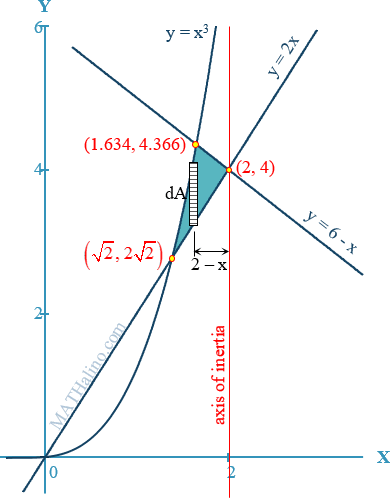# moment of inertia of an area with respect to the line x = 2

2 posts / 0 new
Roydon Jude S. ...moment of inertia of an area with respect to the line x = 2

The moment of inertia bounded by y=x^3 , y=2x , X + y= 6, with respect to x=2 pa help po until Friday. Plus ask ko Lang po ang pinakakapakipakinabang na calculus book for integration applications. Thanks.

Jhun Vert$I_{\text{at }x = 2} = \Sigma \left[ \int_{x_1}^{x_2}} (2 - x)^2 \, dA \right$$I_{\text{at }x = 2} = \int_{\sqrt{2}}^{1.634}} (2 - x)^2(x^3 - 2x) \, dx + \int_{1.634}^2} (2 - x)^2 [ \, (6 - x) - 2x \, ] \, d$

$I_{\text{at }x = 2} = 0.0351 ~ \text{unit}^4$

• Mathematics inside the configured delimiters is rendered by MathJax. The default math delimiters are $$...$$ and $...$ for displayed mathematics, and $...$ and $...$ for in-line mathematics.